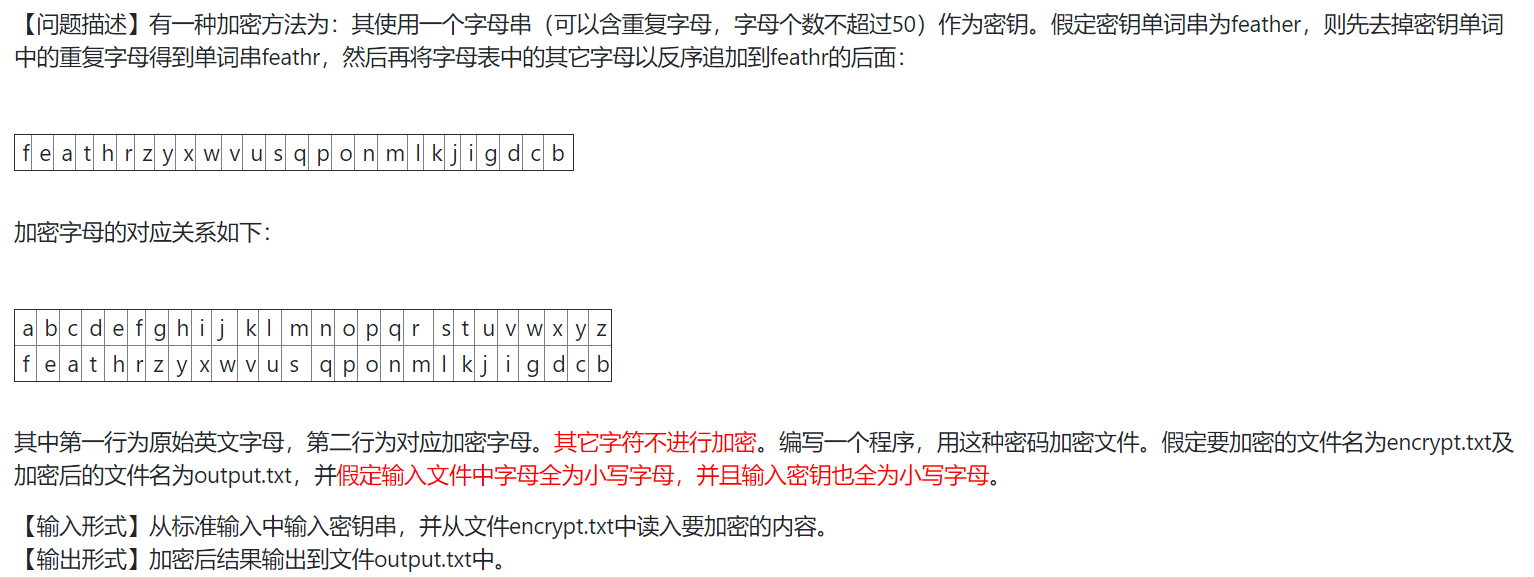2021-06-02 10:05

# python加密文件读取• 点赞
• 写回答
• 关注问题
• 收藏
• 邀请回答

#### 2条回答默认 最新

•已采纳
``````s=input()
zimu="abcdefghijklmnopqrstuvwxyz"
zimu=zimu[::-1]#逆序
t=[0,0,0,0,0,0,0,0,0,0,0,0,0,0,0,0,0,0,0,0,0,0,0,0,0,0,0,0,0,0,0,0,]
with open('encrypt.txt','r')as f:
s1=""
res=""
l= len(s)
for i in range(l):#去重
if(s[i]>='a' and s[i]<='z'):
if t[ord(s[i])-ord('a')]==0:
s1=s1+s[i]
t[ord(s[i])-ord('a')]=1
else:
continue

#print(data[ord(data)-ord('a')])
for i in range(26):
if t[ord(zimu[i])-ord('a')]==0:
s1=s1+zimu[i]
t[ord(zimu[i]) - ord('a')] =1

l= len(data)
for i in range(l):#加密
if(data[i]>='a' and data[i]<='z'):
res=res+s1[ord(data[i])-ord('a')]
else:
res = res + data[i]
with open('output.txt','w')as f:
f.write(res)``````

首先是去重操作，先选一个字母，然后把该字母后面的相同的元素全部变为0，之后在遍历一遍，输出非零字母。然后是反序追加，在去重数组里出现过的字母就不追加，否则就追加，追加完成后变成加密数组。最后是加密，通过加密数组实现就行了。

点赞 评论
•有问必答小助手 2021-06-02 18:41

您好，我是有问必答小助手，您的问题已经有小伙伴解答了，您看下是否解决，可以追评进行沟通哦~

如果有您比较满意的答案 / 帮您提供解决思路的答案，可以点击【采纳】按钮，给回答的小伙伴一些鼓励哦～～